# Data Mining - Decision boundary Visualization

Classifiers create boundaries in instance space. Different classifiers have different biases. You can explore them by visualizing the classification boundaries.

In Weka, the visualization is restricted to numeric attributes, and 2D plots

## Example

• Logistic Regression method produces linear boundary with a gradual transition from one color to another. Logistic regression is a sophisticated way of choosing a linear decision boundary for classification.
• Support Vector Machine method: The resulting plot have no areas of pure color
• Random Forest method, The boundary shapes has a checkered pattern with slightly fuzzy boundaries
Algorithm Boundary Shapes
Logistic Regression Strictly Linear
Knn piecewise linear
support vector machine piecewise linear
Decision tree definitely non-linear

knn decision boundary in any localized region of instance space is linear, determined by the nearest neighbors of the various classes in that region. But the neighbors change when you move around instance space, so the boundary is a set of linear segments that join together.

Support vector machines also produce piecewise linear boundaries.

c4.5 produces decision trees, which create non-linear boundaries in instance space.

Logistic regression is a sophisticated way of producing a good linear decision boundary, which is necessarily simple and therefore less likely to overfit.

support vector machine produce piecewise linear boundaries, but is resilient against overfitting because it relies on a small number of support vectors.

The Logistic classifier (and also meta.ClassificationViaRegression) calculates a linear decision boundary.

The boosting algorithm AdaBoostM1 has a checkered pattern with crisp boundaries

## Documentation / Reference

Recommended PagesData Mining - Decision Tree (DT) Algorithm

Desicion Tree (DT) are supervised Classification algorithms. They are: easy to interpret (due to the tree structure) a boolean function (If each decision is binary ie false or true) Decision trees...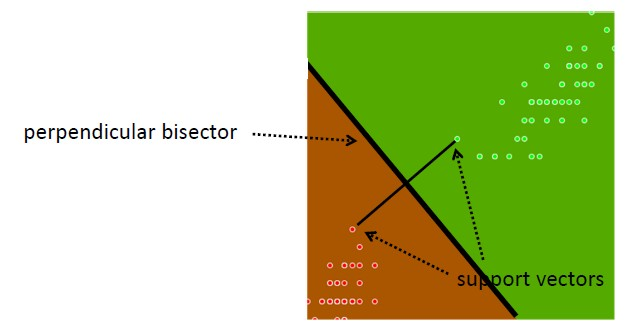Data Mining - Support Vector Machines (SVM) algorithm

A support vector machine is a Classification method. supervised algorithm used for: Classification and Regression (binary and multi-class problem) anomalie detection (one class problem) Supports:...Machine Learning - (Overfitting|Overtraining|Robust|Generalization) (Underfitting)

A learning algorithm is said to overfit if it is: more accurate in fitting known data (ie training data) (hindsight) but less accurate in predicting new data (ie test data) (foresight) Ie the model...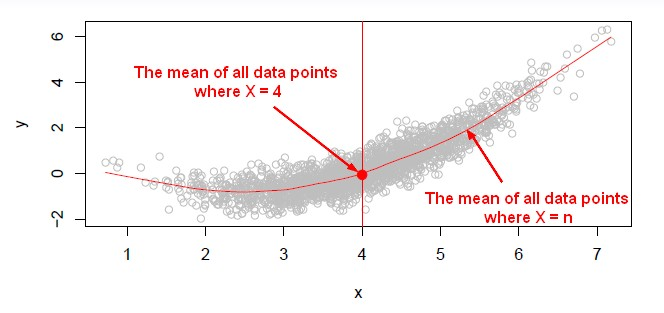Machine Learning - K-Nearest Neighbors (KNN) algorithm - Instance based learning

“Nearest‐neighbor” learning is also known as “Instance‐based” learning. K-Nearest Neighbors, or KNN, is a family of simple: classification and regression algorithms based on Similarity...Statistical Learning - Simple Linear Discriminant Analysis (LDA)

Linear Discriminant Analysis with only one variable (p = 1). For a generalization, see The variance from the distribution of the value when is the same in each of the classes k. It is an important...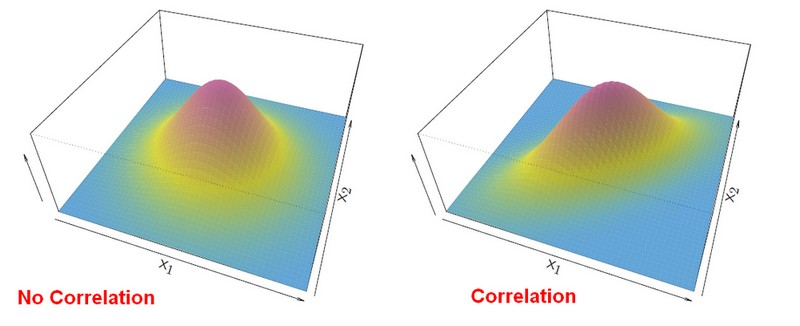Statistics - Fisher (Multiple Linear Discriminant Analysis|multi-variant Gaussian)

multi-variant Gaussians. Fisher has describe first this analysis with his Iris Data Set. A Fisher's linear discriminant analysis or Gaussian LDA measures which centroid from each class is the closest....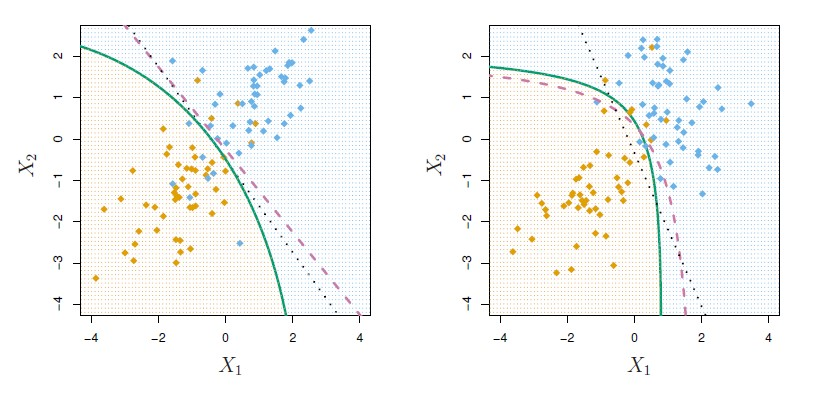Statistics - Quadratic discriminant analysis (QDA)

When the variances of all X are different in each class, the magic of cancellation doesn't occur because when the variances are different in each class, the quadratic terms don't cancel. And therefore...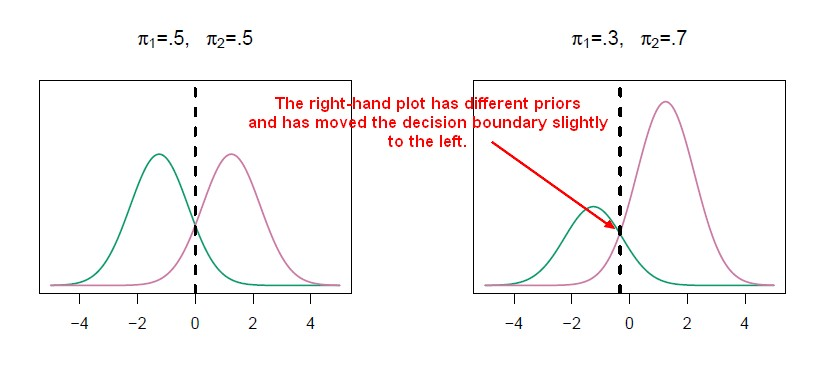Statistics Learning - Discriminant analysis

Discriminant analysis is a classification method. In discriminant analysis, the idea is to: model the distribution of X in each of the classes separately. use what's known as Bayes theorem to flip...